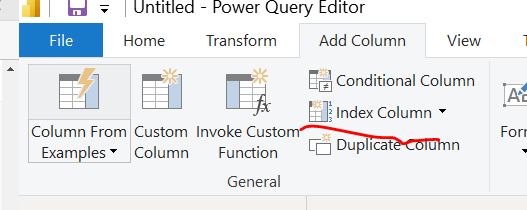cancel
Showing results for
Did you mean:Regular Visitor

## Calculate the value from previous row

I have a table as the following.

Column1    Column2

A                 1000

B                   800

B                   500

B                   300

I would like to create one more column

Column1    Column2   Column3

A                 1000            200

B                   800            300

B                   500            200

B                   300            300

How could I make that?

Thanks.

2 ACCEPTED SOLUTIONSSuper User

you can try to create an index column in PQthen create a column

``````Column =
VAR _next=maxx(FILTER('Table','Table'[Index]=EARLIER('Table'[Index])+1),'Table'[Column2])
return 'Table'[Column2]-_next``````Proud to be a Super User!Super User

Hi,

I assume the order is by column 2.

Please check the below picture and the attached pbix file.``````Column3 CC =
VAR _number =
MAXX (
FILTER ( Data, Data[Column2] < EARLIER ( Data[Column2] ) ),
Data[Column2]
)
RETURN
IF ( NOT ISBLANK ( _number ), Data[Column2] - _number, Data[Column2] )
``````

If this post helps, then please consider accepting it as the solution to help other members find it faster, and give a big thumbs up.

3 REPLIES 3Super User

Hi,

I assume the order is by column 2.

Please check the below picture and the attached pbix file.``````Column3 CC =
VAR _number =
MAXX (
FILTER ( Data, Data[Column2] < EARLIER ( Data[Column2] ) ),
Data[Column2]
)
RETURN
IF ( NOT ISBLANK ( _number ), Data[Column2] - _number, Data[Column2] )
``````

If this post helps, then please consider accepting it as the solution to help other members find it faster, and give a big thumbs up.Regular Visitor

resolved, perfect!Super User

you can try to create an index column in PQthen create a column

``````Column =
VAR _next=maxx(FILTER('Table','Table'[Index]=EARLIER('Table'[Index])+1),'Table'[Column2])
return 'Table'[Column2]-_next``````Proud to be a Super User!